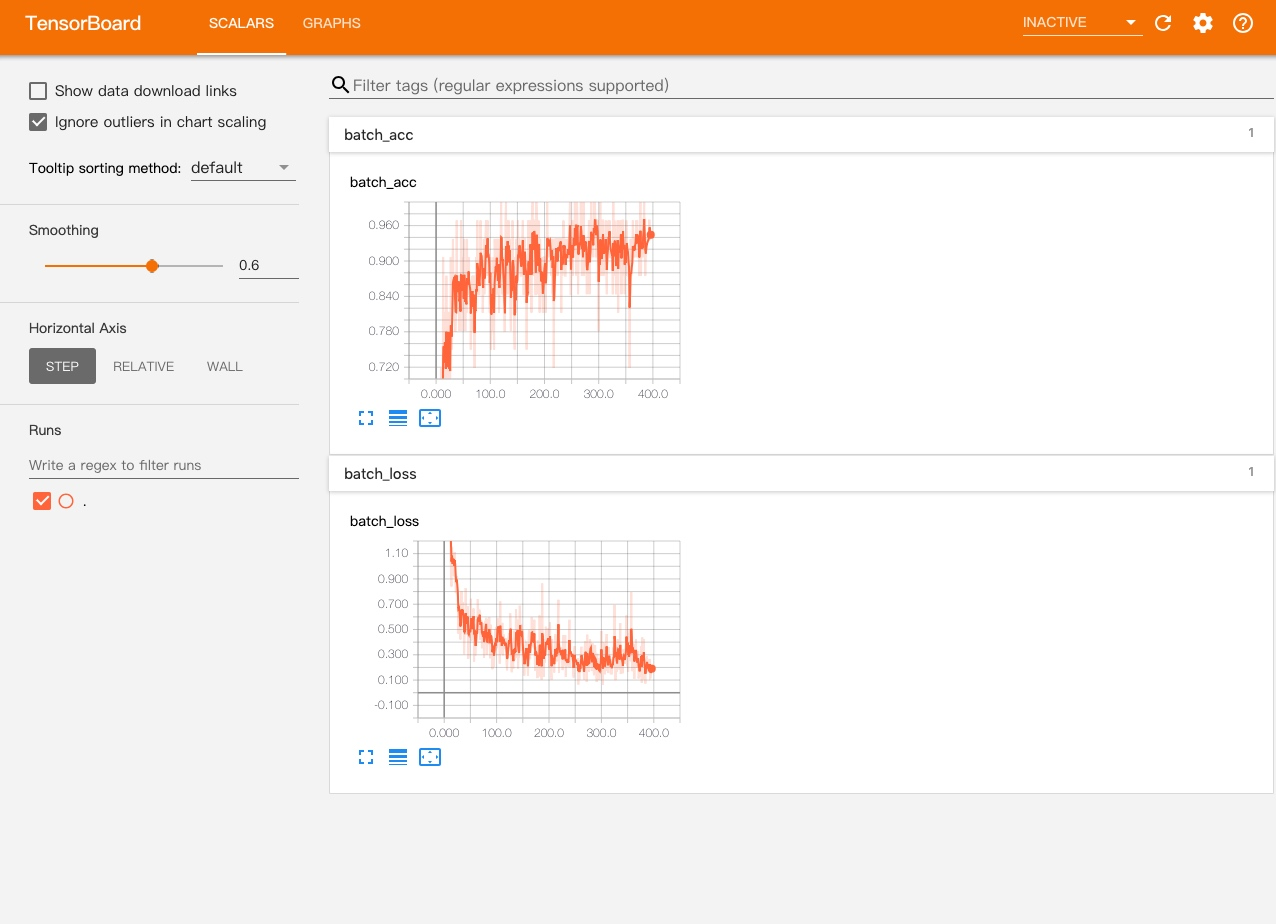# 使用 TensorBoard

def create_model():  model = tf.keras.models.Sequential([  tf.keras.layers.Dense(512, activation=tf.nn.relu, input_shape=(img_rows * img_cols,)),  tf.keras.layers.Dropout(0.2),  tf.keras.layers.Dense(num_classes, activation=tf.nn.softmax)])  model.compile(optimizer='adam',              loss='sparse_categorical_crossentropy',              metrics=['accuracy'])  return modelmodel = create_model()model.summary()log_path = './tf_dir'# 设置 TensorBoard 路径到 tf_dirtb_callback = tf.keras.callbacks.TensorBoard(log_dir=log_path)model.fit(x_train, y_train,          epochs=epochs,          verbose=1,          callbacks=[tb_callback])tensorboard --logdir=<TensorBoard 数据目录>